Courses

# NCERT Solutions (Part- 1)- Algebraic Expressions and Identities Class 8 Notes | EduRev

## Mathematics (Maths) Class 8

Created by: Full Circle

## Class 8 : NCERT Solutions (Part- 1)- Algebraic Expressions and Identities Class 8 Notes | EduRev

The document NCERT Solutions (Part- 1)- Algebraic Expressions and Identities Class 8 Notes | EduRev is a part of the Class 8 Course Mathematics (Maths) Class 8.
All you need of Class 8 at this link: Class 8

Question 1. Give five examples of expressions containing one variable and five examples of expressions containing two variables.

Solution:

(a) Five examples containing one variable are:

(i) x – 4 (ii) 5 – y (iii) 2x + 5 (iv) 10x + 4 (v) 11 – z

(b) Five examples containing two variables are:

(i) 7x – 2y (ii) 5x + 2y – 10 (iii) 6x + z – 2 (iv) 15y – z (v) 9x – 10z

Question 2. Show on the number line x, x – 4, 2x + 1, 3x – 2.

Solution: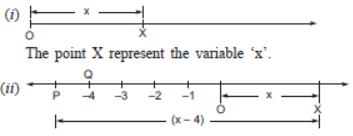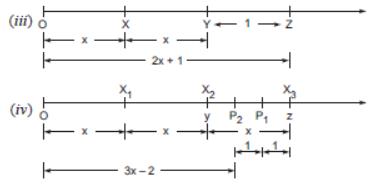Term, Factors and Coefficient

Consider the expression 3x + 7. This expression is containing two terms, i.e. 3x and 7.

Terms are added to form expressions.

Now consider the expression 7x2 – 5xy. This expression contains two terms, i.e. 7x2 and –5xy.

The term 7x2 is a product of 7, x and x. or we say that 7, x and x are factors of the term 7x2.

Also the term –5xy has the factors –5, x and y.

We know that a term is a product of its factors.

The numerical factor of term is called its coefficient. The expression 9xy – 7x has the two terms

9xy and –7x. The coefficient of the term 9xy is 9 and the coefficient of –7x is –7.

Question: Identify the coefficient of each term in the expression x2y2 – 10x2y + 5xy2 – 20.

Solution:

Coefficient of x2y2 is 1.

Coefficient of x2y is –10.

Coefficient of xy2 is 5.

Monomials, Binomials and Polynomials

An expression containting only one term is called monomial.

Examples: 2x, 3y, 4p, q, 3x2, 4y2, 7xy, – 9m, –3, 4xyz, etc.

An expression containing two terms is called binomial.

Examples: a + b, x – y, 2p + 4q, 3xy – 7yz, p2 – 3q2, p2 – 3, a + 3, etc.

An expression containing three terms is called trinomial.

Examples: a + b + c, 4p + 9q – 3r, x+ 4y2 – 3z2, etc.

An expression containing one or more terms with non-zero coefficient of variables having nonnegative exponents is called a polynomial.

Examples: a + b + c + d, 4xy, –5z, 5xyz – 10p, 4x + 3y – 3z, etc.

Question 1. Classify the following polynomials as monomials, binomials, trinomials –z + 5, x + y + z, y + z + 100, ab – ac, 17

Solution:

 Monomials Binomials Trinomials 17 –z + 5ab – ac x + y + zy + z + 100

Question 2. Construct:

(a) 3 binomials with only x as a variable;

Sol:  (a) 1. 5x – 4             2. 3x + 2         3. 10 + 2x

(b) 3 binomials with x and y as variables;

Sol:  (b) 1. 2x + 3y           2. 5x – y         3. x – 3y

(c) 3 monomials with x and y as variables;

Sol:  (c) 1. 2xy                 2. xy               3. –7xy

(d) 2 polynomials with 4 or more terms.

Solution:  (d) 1. 2x3 – x2 + 6x – 8     2. 10 + 7x – 2x2 + 4x3

Question: write two terms which are like

(i) 7xy (ii) 4mn2 (iii) 2l.

Solution:

(i) Two terms like 7xy are: –3xy and 8xy.

(ii) Two terms like 4mn2 are: 6mn2 and –2n2m.

(iii) Two terms like 2l are: 5l and –7b.

Exercise 9.1

Question 1. Identify the terms, their coefficients for each of the following expressions.

(i) 5xyz2 – 3zy      (ii) 1 + x + x2     (iii) 4x2y2 – 4x2y2z2 + z2
(iv) 3 – pq + pr – rp  (v) X/2 + X/2 - xy  (vi) 0.3a – 0.6ab + 0.5b

Solution:

(i) 5xyz2 – 3zy

 Terms 5xyz2 –3zy Coefficients 5 –3

(ii) 1 + x + x2

 Terms 1 +x +x2 Coefficients 1 1 1

(iii) 4x2y2 – 4x2y2z2 + z2

 Terms 4x2y2 –4x2y2z2 + z2 + z2 4 –4 1

(iv) 3 – pq + qr – rp

 Terms 3 –pq + qr – rp Coefficients 3 –1 +1 –1

(v) x/2 +x/2 - xy

 Terms x/2 +y/2 -xy Coefficients 1/2 1/2 -1

(vi) 0.3a – 0.6ab + 0.5b

 Terms 0.3a –0.6ab 0.5b Coefficients 0.3 –0.6 0.5

Question 2. Classify the following polynomials as monomials, binomials, trinomials. Which polynomials do not fit in any of these three categories?

x + y, 1000,   x + x+ x3 + x4,   7 + y + 5x, 2y – 3y2,   2y – 3y2 + 4y3,
5x – 4y + 3xy,   4z – 15z2,   ab + bc + cd + da, pqr,   p2q + pq2, 2p + 2q

Solution:

 Monomials Binomials Trinomials 1000pqr x + y2y – 3y24z – 15z2p2q + pq22p + 2q 7 + y + 5x2y – 3y2 + 4y35x – 4y + 3xy

Following polynomials do not fit in any of these categories:

x + x2 + x3 + x2 [∵ It has 4 terms.]

ab + bc + cd + da [∵ It has 4 terms.]

(i) ab – bc, bc – ca, ca – ab

(ii) a – b + ab, b – c + bc, c – a + ac

(iii) 2p2q2 – 3pq + 4, 5 + 7pq – 3p2q2

(iv) l2 + m2, m2 + n2, n2 + l2, 2lm + 2mn + 2nl

Solution: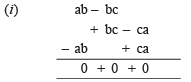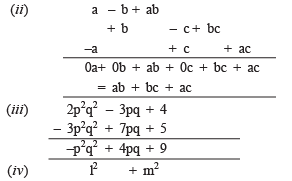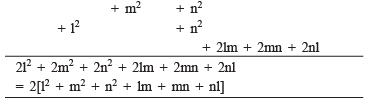Question 4.

(a) Subtract 4a – 7ab + 3b + 12 from 12a – 9ab + 5b – 3.

(b) Subtract 3xy + 5yz – 7zx from 5xy – 2yz – 2zx + 10xyz.

(c) Subtract 4p2q – 3pq + 5pq2 – 8p + 7q – 10

from 18 – 3p – 11q + 5pq – 2pq2 + 5p2q.

Solution: For subtraction we rearrange the terms such that the like terms are in the same column. Then we change the sign of the terms to be subtracted. Next we add the terms.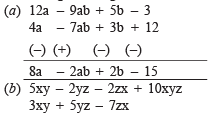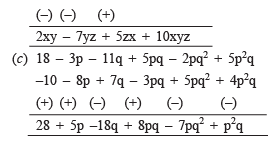Product of Monomials

Note:

I. When we multiply monomials the product is also a monomial

II. We use the rules for exponents and powers when we multiply variables.

Example: Find the product of 2x, 5xy and 7x.

Solution: 2x * 5xy * 7z = (2 * 5 * 7) * (x * xy) * z

= 70 * (x2y 8 z)

= 70 * x2yz = 70x2yz

Question: Find 4x * 5y * 7z. First find 4x * 5y and multiply it by 7z; or first find 5y * 7z and multiply it by 4x. Is the result the same? What do you observe? Does the order in which you carry out the multiplication matter?

Solution: We have:

4x * 5y * 7z = (4x * 5y) * 7z

= 20xy * 7z = 140xyz

Also 4x * 5y * 7z = 4x * (5y 8 7z)

= 4x * 35yz = 140xyz

We observe that

(4x * 5y) * 7x = 4x(5y * 7z)

∴ The product of monomials is associative, i.e. the order in which we multiply the monomials does not matter.

## Mathematics (Maths) Class 8

212 videos|147 docs|48 tests

,

,

,

,

,

,

,

,

,

,

,

,

,

,

,

,

,

,

,

,

,

;